## 3.1 Finite Domain Constraints

Finite domain (FD) constraints have become quite popular and are widely supported. A finite domain variabledenotes an integer. However, we may not know exactly which integer. Typically we only know that it must be one of a finite number of possibilities, e.g. one of 1,2 or 7. This would be represented by a basic constraint: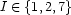Suppose, we have a second FD variable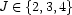. If we unify them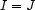, their domains are intersected thus resulting in the conclusion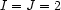. This technique underlies the efficient treatment of agreement: ifencodes the agreement information on one word and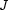on another and the 2 words must agree, then the constraintenforces this agreement.

Denys Duchier
Version 1.2.0 (20010221)